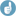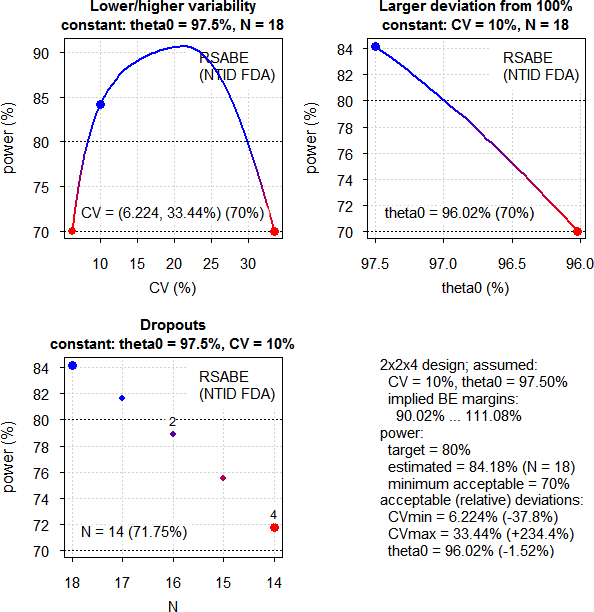PowerTOST: sampleN.NTIDFDA() [Power / Sample Size]

Hi Narra,

» By using the only power and sample size how we can calculate the N for NTI molecules. what about the other parameters.

As with any other reference-scaling method we have to use simulations. The conditions for BE of NTIDs are summarized in this post and the statistical method is given in the FDA’s warfarin-guidance.

Sample size estimations are implemented in function sampleN.NTIDFDA() of the-package PowerTOST since 2013.Example: CVwT = CVwR = 10%, expected T/R-ratio 97.5%, desired (target) power 80%.

library(PowerTOST)
sampleN.NTIDFDA(CV = 0.1)

Gives:

+++++++++++ FDA method for NTIDs ++++++++++++
Sample size estimation
---------------------------------------------
Study design:  2x2x4
log-transformed data (multiplicative model)
1e+05 studies for each step simulated.

alpha  = 0.05, target power = 0.8
CVw(T) = 0.1, CVw(R) = 0.1
True ratio     = 0.975
ABE limits     = 0.8 ... 1.25
Implied scABEL = 0.9002 ... 1.1108
Regulatory settings: FDA
- Regulatory const. = 1.053605
- 'CVcap'           = 0.2142

Sample size search
n     power
14   0.717480
16   0.788690
18   0.841790

Note that in this simple call defaults of the function are applied, namely a full replicate 2-sequence 4-period design (TRTR|RTRT), identical within-subject CVs of T and R, desired (target) power 80%, and T/R 97.5% (the FDA requires for NTIDs tighter content specifications).
Let’s explore what happens if T has a higher variability (15%) than R (10%). Abbreviated output.

print(sampleN.NTIDFDA(CV = c(0.15, 0.1), details = FALSE,
print = FALSE)[8:9], row.names = FALSE)
Sample size Achieved power
32        0.81756

Much higher sample size because it will be more difficult to meet the sWT/sWR criterion.

Note that the FDA wants a 4-period full replicate. If you are concerned about blood loss and/or dropouts you may consider a 3-period full replicate (TRT|RTR). Since that deviates from the guidance, I recommend to initiate a controlled correspondence with the OGD first. Like the first example but for 90% power:

sampleN.NTIDFDA(CV = 0.1, design = "2x2x3", targetpower = 0.9)

+++++++++++ FDA method for NTIDs ++++++++++++
Sample size estimation
---------------------------------------------
Study design:  2x2x3
log-transformed data (multiplicative model)
1e+05 studies for each step simulated.

alpha  = 0.05, target power = 0.9
CVw(T) = 0.1, CVw(R) = 0.1
True ratio     = 0.975
ABE limits     = 0.8 ... 1.25
Implied scABEL = 0.9002 ... 1.1108
Regulatory settings: FDA
- Regulatory const. = 1.053605
- 'CVcap'           = 0.2142

Sample size search
n     power
34   0.894880
36   0.910210

We can also explore deviations from our assumptions (only equal CVs implemented). For the first example, minimum acceptable power 70% (default of the function):

pa.NTIDFDA(CV = 0.1)

Sample size plan RSABE NTID
Design alpha CVwT CVwR theta0 theta1 theta2 Sample size Achieved power Target power
2x2x4  0.05  0.1  0.1  0.975    0.8   1.25          18        0.84179          0.8

Power analysis
CV, theta0 and number of subjects which lead to min. acceptable power of at least 0.7:
CV = (0.0622, 0.3344), theta0= 0.9602
N = 14 (power= 0.7175)Note the interesting behavior of power with various CVs. If the CV gets smaller, limits get tighter and power drops. On the other hand, if the CV increases, we have wider limits and gain power. If the CVwR >21.42% the additional criterion “must pass 80–125%” becomes increasingly important and power drops.

We can also explore which of the three criteria are most important. Again the first example and the estimated sample size 18:

power.NTIDFDA(CV = 0.1, n = 18, details = TRUE)

p(BE)  p(BE-sABEc)    p(BE-ABE) p(BE-sratio)
0.84179      0.85628      1.00000      0.97210

The scaled criterion drives the sample size.

The second example where CVwT > CVwR:

power.NTIDFDA(CV = c(0.15, 0.1), n = 32, details = TRUE)

p(BE)  p(BE-sABEc)    p(BE-ABE) p(BE-sratio)
0.81756      0.92270      1.00000      0.87137

Here the sWT/sWR criterion drives the sample size.

» FDA published a paper* …
» Please provide your valuable suggestion on the same, for my understanding the same.

Hope the above helps.

Dif-tor heh smusma 🖖
Helmut SchützThe quality of responses received is directly proportional to the quality of the question asked. 🚮
Science QuotesIng. Helmut Schütz